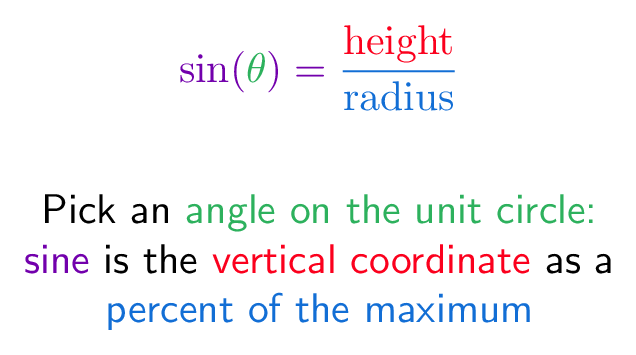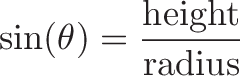# Sine (Unit Circle)

## Colorized Definition\newcommand{\sinefunction}{\color{c1}}
\newcommand{\thetacolor}{\color{c2}}
\newcommand{\opposite}{\color{c3}}
\newcommand{\hypotenuse}{\color{c4}}$\displaystyle{ \sinefunction \sin(\thetacolor{\theta} \sinefunction) = \hypotenuse \frac{\opposite \textrm{height}}{\textrm{radius}} }$

\plain Pick an \thetacolor angle on the unit circle:
\
\sinefunction sine \plain is the \opposite vertical coordinate
\plain as a
\
\hypotenuse percent of the maximum

## Read More - [How to learn Trigonometry Intuitively](https://betterexplained.com/articles/intuitive-trigonometry/) - [Intuitive Understanding of Sine Waves](https://betterexplained.com/articles/intuitive-understanding-of-sine-waves/)